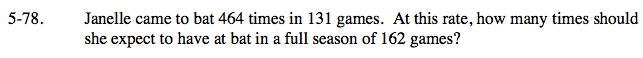Home > CAAC > Chapter 5 > Lesson 5.2.2 > Problem5-78

5-78.

Janelle came to bat 464 times in 131 games. At this rate, how many times should she expect to have at bat in a full season of 162 games? Homework Help ✎$\frac{\text{at bats}}{\text{games}} = \frac{464}{131} = \frac{x}{162}$

$464(162) = 131x$

$75168 = 131x$

$\frac{75168}{131} = x$

Set up a proportion.

573.8015 = x, so about 573 or 574 times.